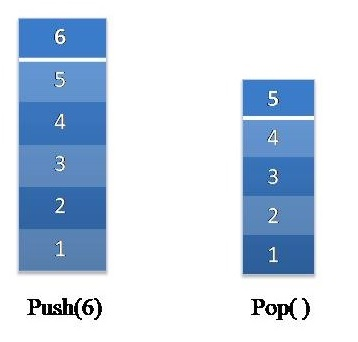# Implement Stack using Linked List in C++

C++ stack implementation using linked list: Here, we are writing a C++ program to implement stack using linked list in C++. By Souvik Saha Last updated : July 31, 2023

To implement a stack using a linked list, basically we need to implement the push() and pop() operations of a stack using linked list.

## Example

Input numbers: 1,3,5,8,4,0

We push the numbers into the stack and whenever it executes a pop() operation, the number is popped out from the stack.## Algorithm

To implement the push() operation:

1. If the Linked list is empty then create a node and point it as head of that Linked List.
2. If the Linked List is not empty then create a node with the input number to be pushed and make it head of the Linked List.

To implement The pop() operation

1. If the Linked List is already empty then do nothing. Output that empty stack.
2. If the Linked List is not empty then delete the node from head.

## C++ implementation

```#include<bits/stdc++.h>
using namespace std;

struct node{
int data;
node* next;
};

//Create a new node
struct node* create_node(int x){
struct node* temp= new node;
temp->data=x;
temp->next=NULL;
return temp;
}

//Enter the node into the linked list
struct node* store=create_node(x);
return;
}
store->next=temp;
}

//pop from the stack
return;
}
while(temp){
cout<<temp->data<<" ";
temp=temp->next;
}
}

int main()
{
struct node* l=NULL;
push(&l,1);
push(&l,2);
push(&l,3);
push(&l,4);
push(&l,5);
push(&l,6);
cout<<"Before the pop operation"<<endl;
print(l);
pop(&l);
pop(&l);
cout<<"\nAfter the pop operation"<<endl;
print(l);
return 0;
}
```

## Output

```Before the pop operation
6 5 4 3 2 1
After the pop operation
4 3 2 1
```